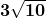## Find the distance between the two points in simplest radical form. (8,−8) and (−1,−5)

Question

Find the distance between the two points in simplest radical form. (8,−8) and (−1,−5)

in progress 0
6 months 2021-07-26T22:29:16+00:00 1 Answers 13 views 0

Solution given: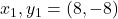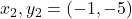Now

Distance between them is:

d=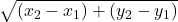d=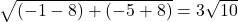Distance between them is